GFG App
Open AppBrowser
Continue

# Reversal algorithm for Array rotation

Given an array arr[] of size N, the task is to rotate the array by d position to the left.

Examples:

Input:  arr[] = {1, 2, 3, 4, 5, 6, 7}, d = 2
Output: 3, 4, 5, 6, 7, 1, 2
Explanation: If the array is rotated by 1 position to the left,
it becomes {2, 3, 4, 5, 6, 7, 1}.
When it is rotated further by 1 position,
it becomes: {3, 4, 5, 6, 7, 1, 2}

Input: arr[] = {1, 6, 7, 8}, d = 3
Output: 8, 1, 6, 7Approach: We have already discussed several methods in this post. The ways discussed there are:

• Using another temporary array.
• Rotating one by one.
• Using a juggling algorithm.

Another Approach (The Reversal Algorithm): Here we will be discussing another method which uses the concept of reversing a part of array. The intuition behind the idea is mentioned below:

Intuition:

If we observe closely, we can see that a group of array elements is changing its position. For example see the following array:
arr[] = {1, 2, 3, 4, 5, 6, 7} and d = 2. The rotated array is {3, 4, 5, 6, 7, 1, 2}

The group having the first two elements is moving to the end of the array. This is like reversing the array.

• But the issue is that if we only reverse the array, it becomes {7, 6, 5, 4, 3, 2, 1}.
• After rotation the elements in the chunks having the first 5 elements {7, 6, 5, 4, 3} and the last 2 elements {2, 1} should be in the actual order as of the initial array [i.e., {3, 4, 5, 6, 7} and {1, 2}]but here it gets reversed.
• So if those blocks are reversed again we get the desired rotated array.

So the sequence of operations is:

• Reverse the whole array
• Then reverse the last ‘d’ elements and
• Then reverse the first (N-d) elements.

As we are performing reverse operations it is also similar to the following sequence:

• Reverse the first ‘d’ elements
• Reverse last (N-d) elements
• Reverse the whole array.

Algorithm: The algorithm can be described with the help of the below pseudocode:

Pseudocode:

Algorithm reverse(arr, start, end):
mid = (start + end)/2
loop from i = start to mid:
swap (arr[i], arr[end-(mid-i+1)])

Algorithm rotate(arr, d, N):
reverse(arr, 1, d) ;
reverse(arr, d + 1, N);
reverse(arr, 1, N);

Illustration:

Follow the illustration below to for  better understanding of the algorithm and intuition:

For example take the array arr[] = {1, 2, 3, 4, 5, 6, 7} and d = 2.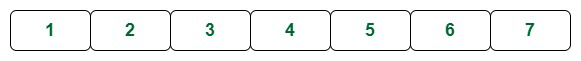Array

The rotated array will look like: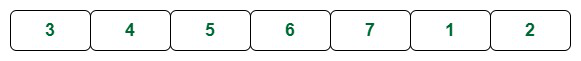Rotated Array

1st Step: Consider the array as a combination of two blocks. One containing the first two elements and the other containing the remaining elements as shown above.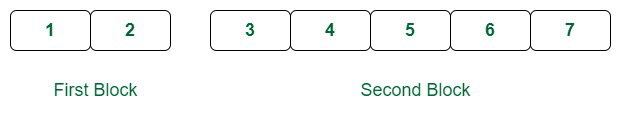Considered 2 blocks

2nd Step: Now reverse the first d elements. It becomes as shown in the image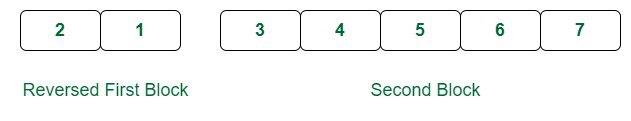Reverse the first K elements

3rd Step: Now reverse the last (N-d) elements. It become as it is shown in the below image: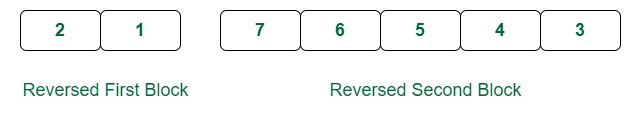Reverse the last (N-K) elements

4th Step: Now the array is the exact reversed form of how it should be if left shifted d times. So reverse the whole array and you will get the required rotated array.The total array is reversed

See that the array is now the same as the rotated array.

Below is the implementation of the above approach:

## C++

 `#include // for reverse function` `#include   // for input/output operations` `#include     // for vector container` `using` `namespace` `std;`   `// Function to rotate an array by k elements to the right` `void` `rotateArray(vector<``int``>& arr, ``int` `k)` `{` `    ``// Find the size of the array` `    ``int` `n = arr.size();`   `    ``// Mod k with the size of the array` `    ``// To handle the case where k is greater than the size of the array` `    ``k %= n;`   `    ``// Reverse the entire array` `    ``reverse(arr.begin(), arr.end());`   `    ``// Reverse the first k elements` `    ``reverse(arr.begin(), arr.begin() + k);`   `    ``// Reverse the remaining n-k elements` `    ``reverse(arr.begin() + k, arr.end());` `}`   `int` `main()` `{` `    ``// Initialize the array` `    ``vector<``int``> arr = { 1, 2, 3, 4, 5 };`   `    ``// Number of elements to rotate to the right` `    ``int` `k = 2;`   `    ``// Call the rotateArray function to rotate the array` `    ``rotateArray(arr, k);`   `    ``// Print the rotated array` `    ``for` `(``int` `i : arr) {` `        ``cout << i << ``" "``;` `    ``}`   `    ``// Return 0 to indicate successful termination of the program` `    ``return` `0;` `}`

## C++

 `// C++ program for reversal algorithm` `// of array rotation`   `#include ` `using` `namespace` `std;`   `// Function to reverse arr[] ` `// from index start to end` `void` `reverseArray(``int` `arr[], ``int` `start, ``int` `end)` `{` `    ``while` `(start < end) {` `        ``int` `temp = arr[start];` `        ``arr[start] = arr[end];` `        ``arr[end] = temp;` `        ``start++;` `        ``end--;` `    ``}` `}`   `// Function to left rotate arr[] of size n by d` `void` `leftRotate(``int` `arr[], ``int` `d, ``int` `n)` `{` `    ``if` `(d == 0)` `        ``return``;` `    `  `    ``// In case the rotating factor is` `    ``// greater than array length` `    ``d = d % n;`   `    ``reverseArray(arr, 0, d - 1);` `    ``reverseArray(arr, d, n - 1);` `    ``reverseArray(arr, 0, n - 1);` `}`   `// Function to print an array` `void` `printArray(``int` `arr[], ``int` `size)` `{` `    ``for` `(``int` `i = 0; i < size; i++)` `        ``cout << arr[i] << ``" "``;` `}`   `// Driver code` `int` `main()` `{` `    ``int` `arr[] = { 1, 2, 3, 4, 5, 6, 7 };` `    ``int` `N = ``sizeof``(arr) / ``sizeof``(arr);` `    ``int` `d = 2;`   `    ``// Function call` `    ``leftRotate(arr, d, N);` `    ``printArray(arr, N);` `    ``return` `0;` `}`

## C

 `// C/C++ program for reversal algorithm of array rotation` `#include `   `/*Utility function to print an array */` `void` `printArray(``int` `arr[], ``int` `size);`   `/* Utility function to reverse arr[] from start to end */` `void` `reverseArray(``int` `arr[], ``int` `start, ``int` `end);`   `/* Function to left rotate arr[] of size n by d */` `void` `leftRotate(``int` `arr[], ``int` `d, ``int` `n)` `{`   `    ``if` `(d == 0)` `        ``return``;` `    ``// in case the rotating factor is` `    ``// greater than array length` `    ``d = d % n;`   `    ``reverseArray(arr, 0, d - 1);` `    ``reverseArray(arr, d, n - 1);` `    ``reverseArray(arr, 0, n - 1);` `}`   `/*UTILITY FUNCTIONS*/` `/* function to print an array */` `void` `printArray(``int` `arr[], ``int` `size)` `{` `    ``int` `i;` `    ``for` `(i = 0; i < size; i++)` `        ``printf``(``"%d "``, arr[i]);` `}`   `/*Function to reverse arr[] from index start to end*/` `void` `reverseArray(``int` `arr[], ``int` `start, ``int` `end)` `{` `    ``int` `temp;` `    ``while` `(start < end) {` `        ``temp = arr[start];` `        ``arr[start] = arr[end];` `        ``arr[end] = temp;` `        ``start++;` `        ``end--;` `    ``}` `}`   `/* Driver program to test above functions */` `int` `main()` `{` `    ``int` `arr[] = { 1, 2, 3, 4, 5, 6, 7 };` `    ``int` `n = ``sizeof``(arr) / ``sizeof``(arr);` `    ``int` `d = 2;`   `    ``leftRotate(arr, d, n);` `    ``printArray(arr, n);` `    ``return` `0;` `}`

## Java

 `// Java program for reversal algorithm of array rotation` `import` `java.io.*;`   `class` `LeftRotate {` `    ``/* Function to left rotate arr[] of size n by d */` `    ``static` `void` `leftRotate(``int` `arr[], ``int` `d)` `    ``{`   `        ``if` `(d == ``0``)` `            ``return``;`   `        ``int` `n = arr.length;` `        ``// in case the rotating factor is` `        ``// greater than array length` `        ``d = d % n;` `        ``reverseArray(arr, ``0``, d - ``1``);` `        ``reverseArray(arr, d, n - ``1``);` `        ``reverseArray(arr, ``0``, n - ``1``);` `    ``}`   `    ``/*Function to reverse arr[] from index start to end*/` `    ``static` `void` `reverseArray(``int` `arr[], ``int` `start, ``int` `end)` `    ``{` `        ``int` `temp;` `        ``while` `(start < end) {` `            ``temp = arr[start];` `            ``arr[start] = arr[end];` `            ``arr[end] = temp;` `            ``start++;` `            ``end--;` `        ``}` `    ``}`   `    ``/*UTILITY FUNCTIONS*/` `    ``/* function to print an array */` `    ``static` `void` `printArray(``int` `arr[])` `    ``{` `        ``for` `(``int` `i = ``0``; i < arr.length; i++)` `            ``System.out.print(arr[i] + ``" "``);` `    ``}`   `    ``/* Driver program to test above functions */` `    ``public` `static` `void` `main(String[] args)` `    ``{` `        ``int` `arr[] = { ``1``, ``2``, ``3``, ``4``, ``5``, ``6``, ``7` `};` `        ``int` `n = arr.length;` `        ``int` `d = ``2``;`   `        ``leftRotate(arr, d); ``// Rotate array by d` `        ``printArray(arr);` `    ``}` `}` `/*This code is contributed by Devesh Agrawal*/`

## Python3

 `# Python program for reversal algorithm of array rotation`   `# Function to reverse arr[] from index start to end`     `def` `reverseArray(arr, start, end):` `    ``while` `(start < end):` `        ``temp ``=` `arr[start]` `        ``arr[start] ``=` `arr[end]` `        ``arr[end] ``=` `temp` `        ``start ``+``=` `1` `        ``end ``=` `end``-``1`   `# Function to left rotate arr[] of size n by d`     `def` `leftRotate(arr, d):`   `    ``if` `d ``=``=` `0``:` `        ``return` `    ``n ``=` `len``(arr)` `    ``# in case the rotating factor is` `    ``# greater than array length` `    ``d ``=` `d ``%` `n` `    ``reverseArray(arr, ``0``, d``-``1``)` `    ``reverseArray(arr, d, n``-``1``)` `    ``reverseArray(arr, ``0``, n``-``1``)`   `# Function to print an array`     `def` `printArray(arr):` `    ``for` `i ``in` `range``(``0``, ``len``(arr)):` `        ``print` `(arr[i],end``=``' '``)`     `# Driver function to test above functions` `arr ``=` `[``1``, ``2``, ``3``, ``4``, ``5``, ``6``, ``7``]` `n ``=` `len``(arr)` `d ``=` `2`   `leftRotate(arr, d)  ``# Rotate array by 2` `printArray(arr)`   `# This code is contributed by Devesh Agrawal`

## C#

 `// C# program for reversal algorithm` `// of array rotation` `using` `System;`   `class` `GFG {` `    ``/* Function to left rotate arr[]` `    ``of size n by d */` `    ``static` `void` `leftRotate(``int``[] arr, ``int` `d)` `    ``{`   `        ``if` `(d == 0)` `            ``return``;` `        ``int` `n = arr.Length;` `          ``// in case the rotating factor is` `        ``// greater than array length` `        ``d = d % n;` `        ``reverseArray(arr, 0, d - 1);` `        ``reverseArray(arr, d, n - 1);` `        ``reverseArray(arr, 0, n - 1);` `    ``}`   `    ``/* Function to reverse arr[] from` `    ``index start to end*/` `    ``static` `void` `reverseArray(``int``[] arr, ``int` `start,` `                             ``int` `end)` `    ``{` `        ``int` `temp;` `        ``while` `(start < end) {` `            ``temp = arr[start];` `            ``arr[start] = arr[end];` `            ``arr[end] = temp;` `            ``start++;` `            ``end--;` `        ``}` `    ``}`   `    ``/*UTILITY FUNCTIONS*/` `    ``/* function to print an array */` `    ``static` `void` `printArray(``int``[] arr)` `    ``{` `        ``for` `(``int` `i = 0; i < arr.Length; i++)` `            ``Console.Write(arr[i] + ``" "``);` `    ``}`   `    ``// Driver code` `    ``public` `static` `void` `Main()` `    ``{` `        ``int``[] arr = { 1, 2, 3, 4, 5, 6, 7 };` `        ``int` `n = arr.Length;` `        ``int` `d = 2;`   `        ``leftRotate(arr, d); ``// Rotate array by 2` `        ``printArray(arr);` `    ``}` `}`   `// This code is contributed by Sam007`

## PHP

 ``

## Javascript

 ``

Output

`3 4 5 6 7 1 2 `

Time Complexity: O(N)
Auxiliary Space: O(1)

Another Method :-  Using C++ STL  reverse

## C++

 `#include // for reverse function` `#include   // for input/output operations` `#include     // for vector container` `using` `namespace` `std;`   `// Function to rotate an array by k elements to the right` `void` `rotateArray(vector<``int``>& arr, ``int` `k)` `{` `    ``// Find the size of the array` `    ``int` `n = arr.size();`   `    ``// Mod k with the size of the array` `    ``// To handle the case where k is greater than the size of the array` `    ``k %= n;`   `    ``// Reverse the entire array` `    ``reverse(arr.begin(), arr.end());`   `    ``// Reverse the first k elements` `    ``reverse(arr.begin(), arr.begin() + k);`   `    ``// Reverse the remaining n-k elements` `    ``reverse(arr.begin() + k, arr.end());` `}`   `int` `main()` `{` `    ``// Initialize the array` `    ``vector<``int``> arr = { 1, 2, 3, 4, 5 };`   `    ``// Number of elements to rotate to the right` `    ``int` `k = 2;`   `    ``// Call the rotateArray function to rotate the array` `    ``rotateArray(arr, k);`   `    ``// Print the rotated array` `    ``for` `(``int` `i : arr) {` `        ``cout << i << ``" "``;` `    ``}`   `    ``// Return 0 to indicate successful termination of the program` `    ``return` `0;` `}`

## Java

 `import` `java.util.ArrayList;` `import` `java.util.Collections;`   `public` `class` `Main {` `    ``public` `static` `void` `main(String[] args)` `    ``{` `        ``// Initialize the array` `        ``ArrayList arr = ``new` `ArrayList<>();` `        ``arr.add(``1``);` `        ``arr.add(``2``);` `        ``arr.add(``3``);` `        ``arr.add(``4``);` `        ``arr.add(``5``);` `        ``// Number of elements to rotate to the right` `        ``int` `k = ``2``;`   `        ``// Call the rotateArray function to rotate the array` `        ``rotateArray(arr, k);`   `        ``// Print the rotated array` `        ``for` `(``int` `i : arr) {` `            ``System.out.print(i + ``" "``);` `        ``}` `    ``}`   `    ``// Function to rotate an array by k elements to the` `    ``// right` `    ``public` `static` `void` `rotateArray(ArrayList arr,` `                                   ``int` `k)` `    ``{` `        ``// Find the size of the array` `        ``int` `n = arr.size();`   `        ``// Mod k with the size of the array` `        ``// To handle the case where k is greater than the` `        ``// size of the array` `        ``k %= n;`   `        ``// Reverse the entire array` `        ``Collections.reverse(arr);`   `        ``// Reverse the first k elements` `        ``for` `(``int` `i = ``0``; i < k / ``2``; i++) {` `            ``int` `temp = arr.get(i);` `            ``arr.set(i, arr.get(k - i - ``1``));` `            ``arr.set(k - i - ``1``, temp);` `        ``}`   `        ``// Reverse the remaining n-k elements` `        ``for` `(``int` `i = k; i < (n + k) / ``2``; i++) {` `            ``int` `temp = arr.get(i);` `            ``arr.set(i, arr.get(n + k - i - ``1``));` `            ``arr.set(n + k - i - ``1``, temp);` `        ``}` `    ``}` `}`

## Python3

 `# Function to rotate an array by k elements to the right` `def` `rotateArray(arr, k):` `    ``# Find the size of the array` `    ``n ``=` `len``(arr);`   `    ``# Mod k with the size of the array` `    ``# To handle the case where k is greater than the size of the array` `    ``k ``%``=` `n;`   `    ``# Reverse the entire array` `    ``arr[``0``:n] ``=` `arr[``0``:n][::``-``1``]`   `    ``# Reverse the first k elements` `    ``arr[``0``:k] ``=` `arr[``0``:k][::``-``1``]`   `    ``# Reverse the remaining n-k elements` `    ``arr[k:n] ``=` `arr[k:n][::``-``1``]`   `# Initialize the array` `arr ``=` `[ ``1``, ``2``, ``3``, ``4``, ``5` `];`   `# Number of elements to rotate to the right` `k ``=` `2``;`   `# Call the rotateArray function to rotate the array` `rotateArray(arr, k);`   `# Print the rotated array` `for` `i ``in` `range``(``0``,``len``(arr)):` `    ``print``(arr[i], end``=` `" "``);`

## Javascript

 `// Define the function to rotate an array` `function` `rotateArray(arr, k) {` `// Find the length of the array` `const n = arr.length;`   `// Mod k with the length of the array` `// To handle the case where k is greater than the` `// length of the array` `k %= n;`   `// Reverse the entire array` `arr.reverse();`   `// Reverse the first k elements` `for` `(let i = 0; i < k / 2; i++) {` `const temp = arr[i];` `arr[i] = arr[k - i - 1];` `arr[k - i - 1] = temp;` `}`   `// Reverse the remaining n-k elements` `for` `(let i = k; i < (n + k) / 2; i++) {` `const temp = arr[i];` `arr[i] = arr[n + k - i - 1];` `arr[n + k - i - 1] = temp;` `}` `}`   `// Initialize the array` `const arr = [1, 2, 3, 4, 5];`   `// Number of elements to rotate to the right` `const k = 2;`   `// Call the rotateArray function to rotate the array` `rotateArray(arr, k);`   `// Print the rotated array` `console.log(arr.join(``' '``));`

## C#

 `using` `System;` `using` `System.Linq;`   `namespace` `ArrayRotation {` `class` `Program {` `    ``static` `void` `Main(``string``[] args)` `    ``{` `        ``// Initialize the array` `        ``int``[] arr = { 1, 2, 3, 4, 5 };`   `        ``// Number of elements to rotate to the right` `        ``int` `k = 2;`   `        ``// Call the RotateArray function to rotate the array` `        ``RotateArray(``ref` `arr, k);`   `        ``// Print the rotated array` `        ``Console.WriteLine(``string``.Join(``" "``, arr));`   `        ``// Wait for the user to end the program` `        ``Console.ReadKey();` `    ``}`   `    ``// Function to rotate an array by k elements to the` `    ``// right` `    ``static` `void` `RotateArray(``ref` `int``[] arr, ``int` `k)` `    ``{` `        ``// Find the size of the array` `        ``int` `n = arr.Length;`   `        ``// Mod k with the size of the array` `        ``// To handle the case where k is greater than the` `        ``// size of the array` `        ``k %= n;`   `        ``// Reverse the first n-k elements` `        ``Array.Reverse(arr, 0, n - k);`   `        ``// Reverse the remaining k elements` `        ``Array.Reverse(arr, n - k, k);`   `        ``// Reverse the entire array` `        ``Array.Reverse(arr);` `    ``}` `}` `}`

Output

`4 5 1 2 3 `

Time Complexity: O(N)
Auxiliary Space: O(1)

My Personal Notes arrow_drop_up